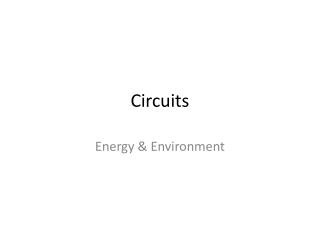# Circuits - PowerPoint PPT PresentationDownload PresentationCircuits

Circuits
Download Presentation## Circuits

- - - - - - - - - - - - - - - - - - - - - - - - - - - E N D - - - - - - - - - - - - - - - - - - - - - - - - - - -
##### Presentation Transcript

1. Circuits Energy & Environment

2. Simple Circuit What symbols are shown here and what do they do? Connect circuit together Wire Power Source -ve Electricity to Light Purpose G Battery +ve Lamp Switch Controlling Device Safety Device Fuse

3. Closed Circuit Where is there potential difference & where is there resistance? For current to flow round circuit, must be a connecting path Some resistance -ve Potential difference G Potential difference +ve Low resistance

4. Open circuit Where is there potential difference & where is there resistance? Current stops as doesn’t have a complete path No power used -ve G Very high resistance +ve Examples of open circuit

5. Short Circuit Why does the current chose to go this way? Circuits are protected against excessive current by fuses Some resistance -ve Almost no resistance G +ve Results in excessive current Which can cause overheating and damage.

6. Series Circuit Where could we put this bulb if we want to add it in series? -ve G +ve

7. Series Circuit: Current B =4 amps -ve In a series circuit the current is the same across the circuit G A =4 amps Itotal=I1=I2=I3 +ve Current through switch=4 amps If the current through the switch is 4 amps, what is the current through the light bulb A and B?

8. Series Circuit: Voltage The potential difference in EMF across two points is called the potential difference. In a series circuit the voltage is the sum of the EMF This side This side A -ve G B =6 volts 12 volts EMF= 12 volts 12 volts=6 +6 +ve A =6 volts (EMF)vtotal=V1+V2+V3 B

9. Series Circuit: Voltage What happens when we add another bulb? What is the voltage of each identical bulb. In a series circuit the voltage is the sum of the EMF This side This side A -ve =4 volts C G B =4 volts 12 volts EMF= 12 volts 12 volts +ve 12 volts= 3 x light bulbs Light bulb = 12 volts 3 =4 volts each A =4 volts (EMF)vtotal=V1+V2+V3 B

10. Series Circuits If this bulb blows what happens to the other bulbs? The circuit is open so there is no path for the current to travel. If current=0 Power=0 As power=EMF x Current

11. Parallel Circuit Where could we put this bulb if we want to add it in parallel? -ve G +ve

12. Parallel Circuit: Current In a parallel circuit the current is split up throughout the different branches B =2 amps -ve Itotal=I1+I2+I3 A =2 amps G +ve Current through switch=4 amps If the current through the switch is 4 amps, what is the current through the identical light bulbs A and B?

13. Parallel Circuit: Voltage The potential difference in EMF across two points is called the potential difference. Voltage in parallel circuits is equal across branches This side This side A A A 1.5 volts -ve Voltage =1.5 volts G 1.5 volts 1.5 volts 1.5 volts +ve B B B

14. Parallel Circuit: Voltage Voltage in parallel circuits is equal What happens when we add another bulb? This side A A A A 1.5 volts 1.5 volts 1.5 volts -ve Voltage =1.5 volts G 1.5 volts +ve B B B B (EMF)vtotal=V1=V2=V3

15. Parallel Circuits If this bulb blows what happens to the other bulbs? The circuit for that bulb is open but the circuit for the other two bulbs is ok